## generative-models

### How to create non-square images with DALL-E 2

The DALL-E API from OpenAI is a very powerful image generation tool. However one limitation is that it only outputs square images. In this tutorial we will learn how to generate images of arbitrary dimension such as portraits and landscapes.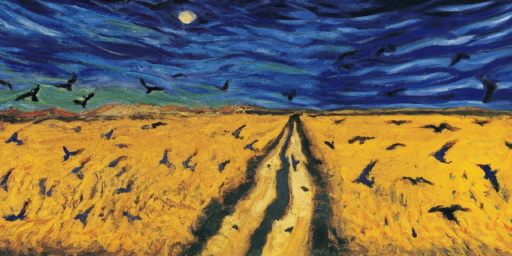### PixelCNN

The PixelRNN family of auto-regressive architectures consists of models that learn to generate images a pixel at a time. In this post we will focus on the PixelCNN model which is based on the same principles but uses convolutional layers instead of recurrent layers. It is simpler to implement and once we have grasped the core concepts, it is straightforward to understand the recurrent versions.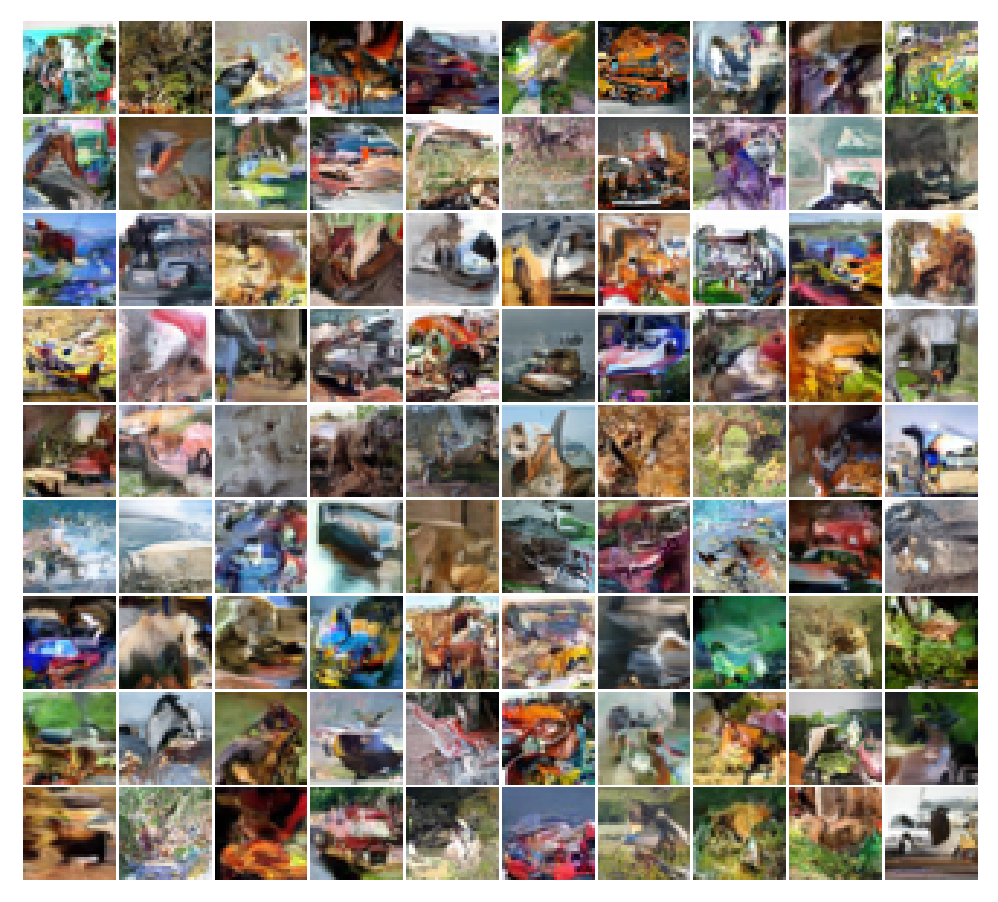### Implementing the Fréchet Inception distance

A popular metric for evaluating image generation models is the Fréchet Inception Distance (FID). Like the Inception score, it is computed on the embeddings from an Inception model. But unlike the Inception score, it makes use of the true images as well as the generated ones. In the post we will learn how to implement it in PyTorch.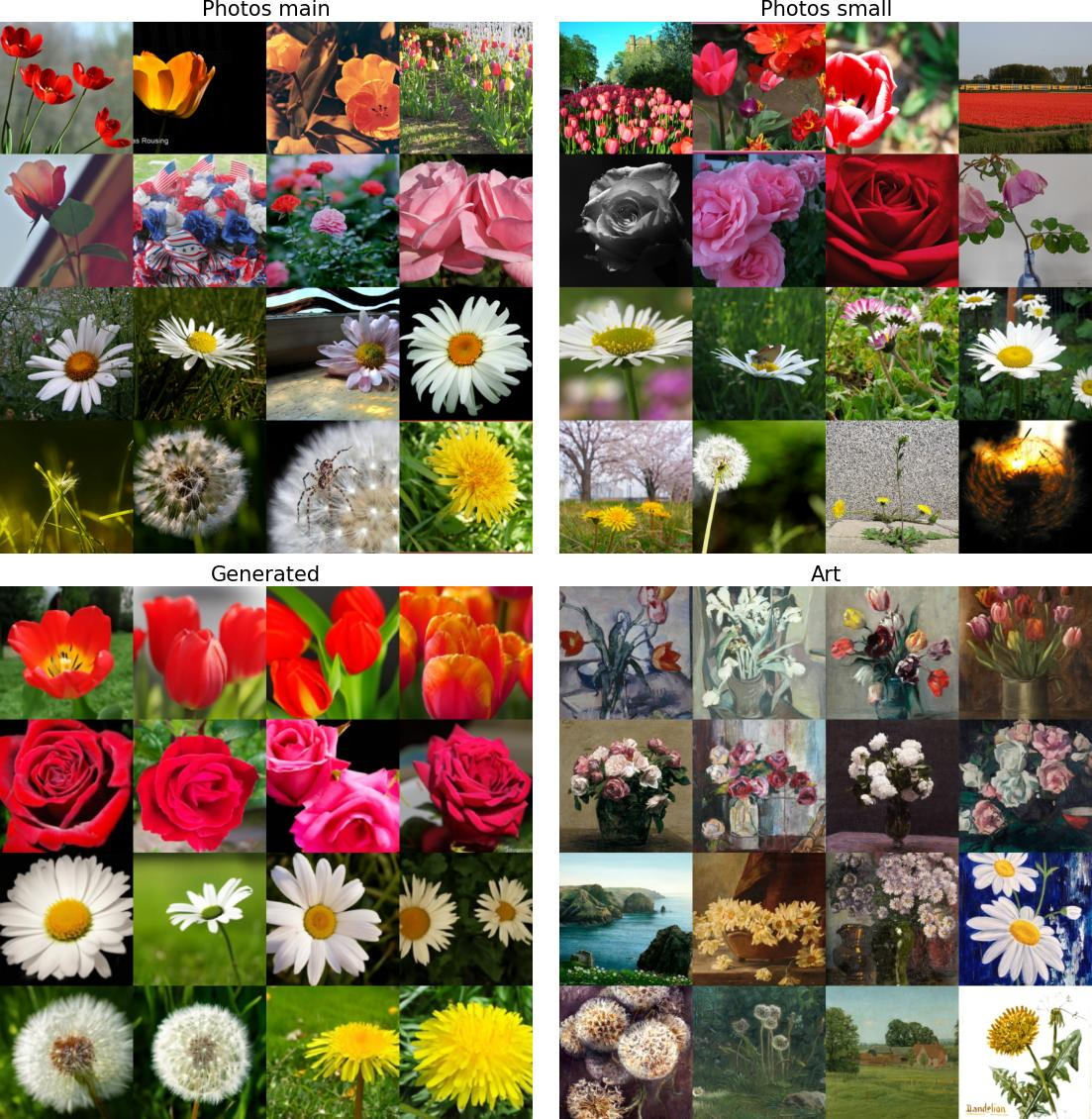### Speeding Up Diffusion Sampling

An important disadvantage with diffusion models is that a large number forward passes - typically 1000 or more - are needed to generate samples, making inference slow and expensive. In this post we will look at a couple of different methods that have been developed to speed up inference.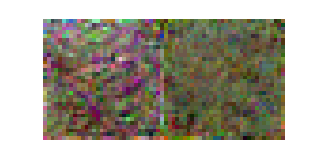### An Introduction to Diffusion

In this blog we will learn about the basic diffusion-based generative model introduced in Denoising Diffusion Probabilistic Models (DDPM). This post will cover the basics at a relatively high level but along the way there will be optional exercises that will help you to delve deeper into the mathematical details.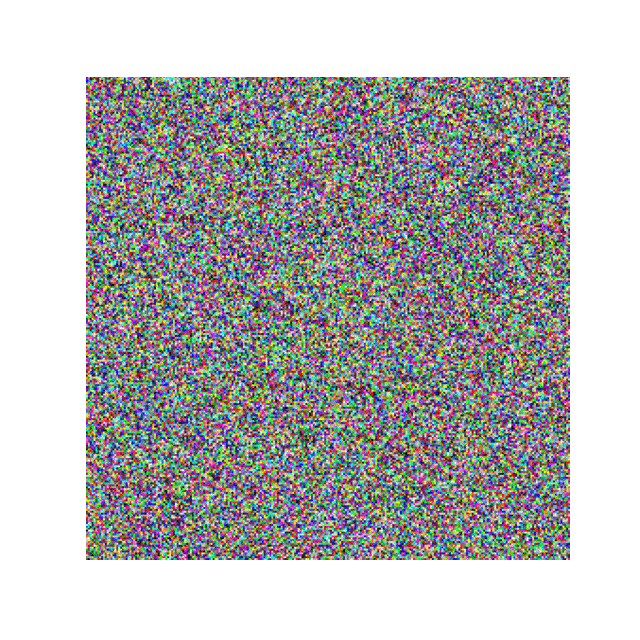### A quick overview of GLIDE

You have probably come across DALL-E 2 a large-scale image generation model from OpenAI. If not, you can read about it in their blog. DALL-E 2 uses a class of models known as diffusion models. The DALL-E 2 paper does not go into a lot of detail about the model architecture since it mostly extends an earlier architecture GLIDE. This article provides a brief introduction to the GLIDE architecture.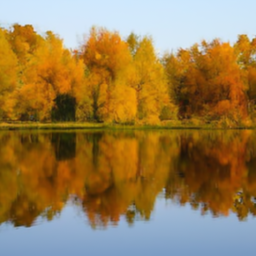## Introduction

In the previous post we motivated the idea of a Variational Auto Encoder. Here we will have a go at implementing a very simple model to get a sense of all the components steps involved.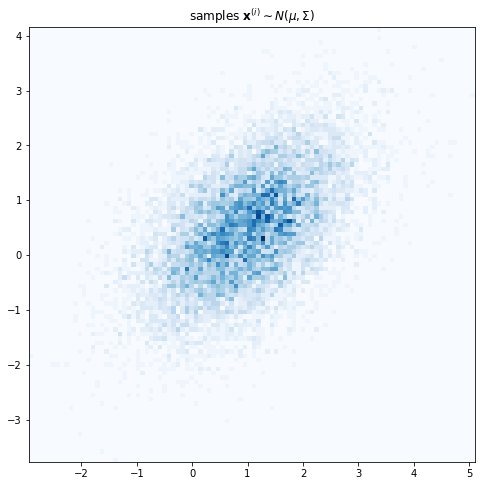### Variational Auto-Encoder - Part 1 / Introduction

Consider this painting by Vincent Van Gogh known as “Wheatfield with Crows”. Suppose you want to learn a probability distribution over Van Gogh’s paintings. What the computer or a deep learning algorithm sees when it looks at this painting is a grid of RGB pixel intensities. So one approach might be to model the data as a sequence of individual pixels. Yet that is manifestly not how the artist has constructed the painting.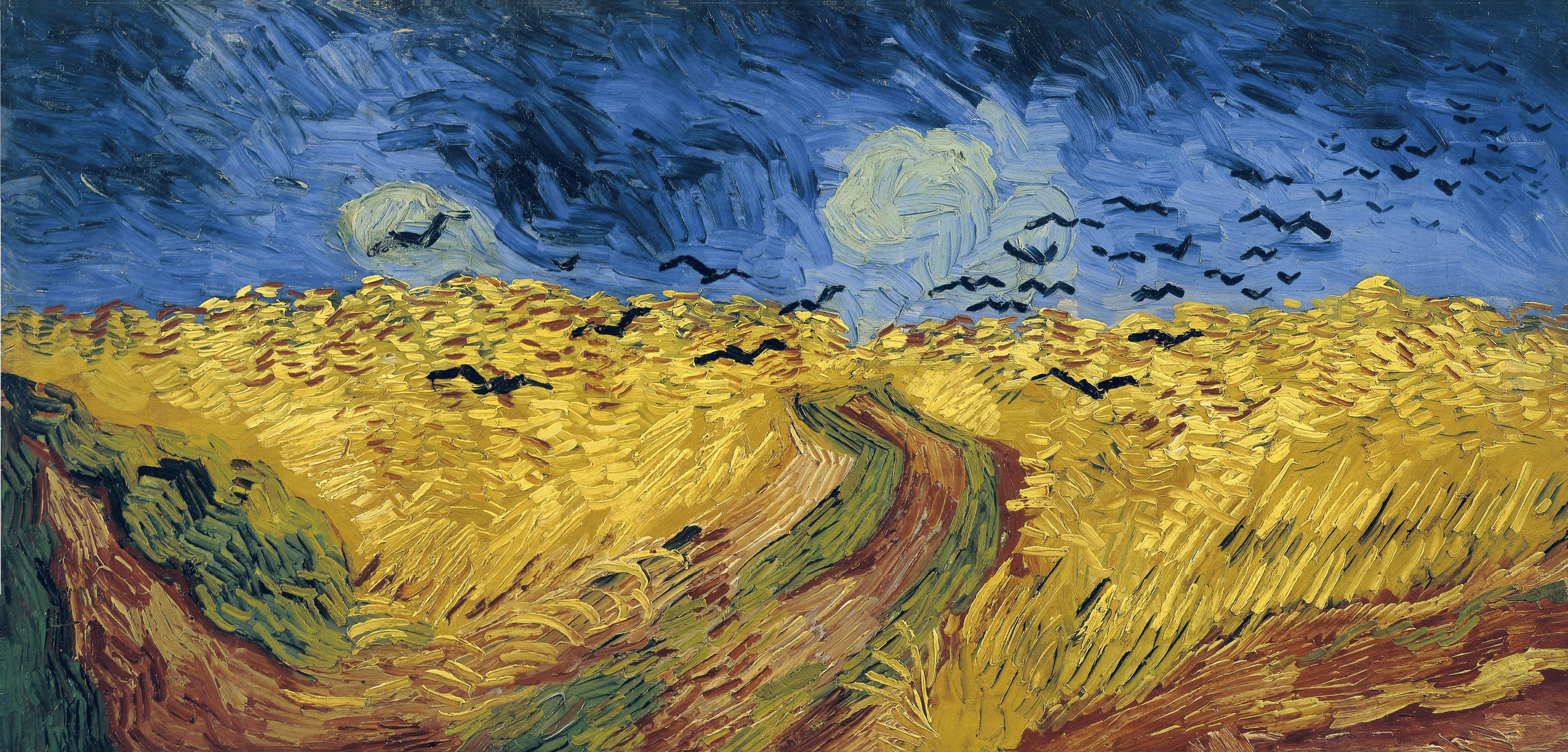## rl

### First Visit Monte Carlo Prediction for Blackjack (RL S&B Example 5.1)

In this tutorial we will demonstrate how to implement Example 5.1: Blackjack from Reinforcement Learning (Sutton and Barto).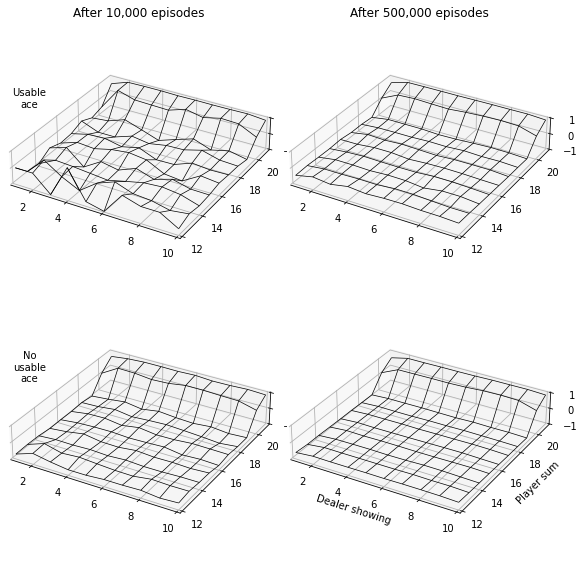### Strassen’s algorithm for matrix multiplication

This is the first of a planned series of blogs covering background topics for DeepMind’s AlphaTensor paper. In this post we will cover Strassen’s algorithm for matrix multiplication.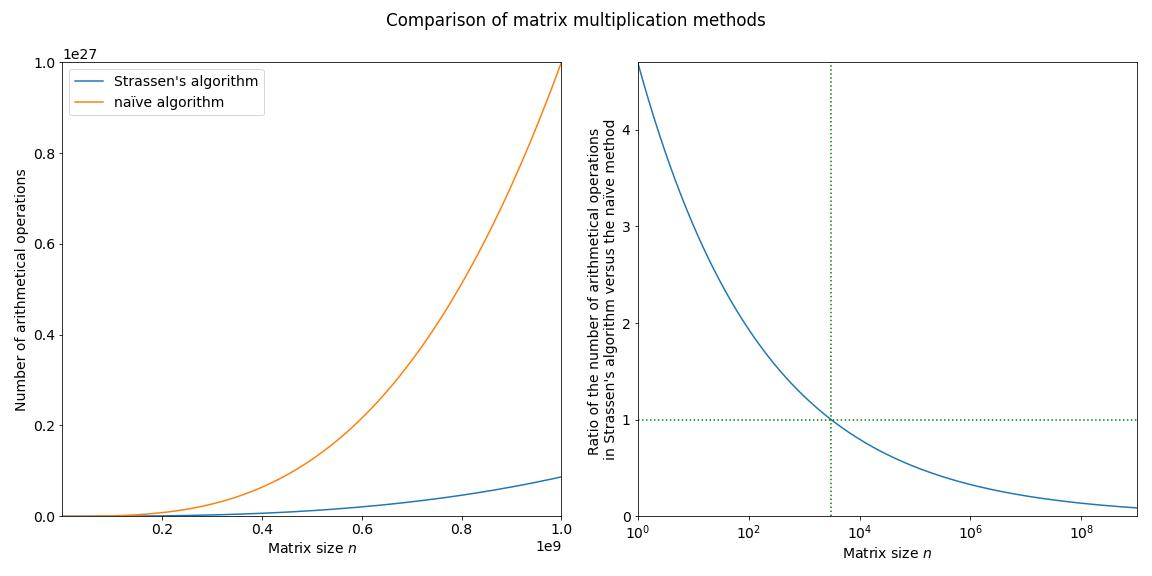### REINFORCE on the short-corridor gridworld, Part 2 (RL S&B Chapter 13)

In the previous post we introducted the short-corridor gridworld with switched actions. We found an analytical solution for the probability for moving right. Now we will use the REINFORCE algorithm to solve this problem.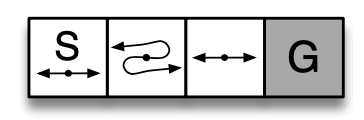### REINFORCE on the short-corridor gridworld, Part 1 (RL S&B Chapter 13)

In this tutorial we will demonstrate how to implement Example 13.1 of Reinforcement Learning (Sutton and Barto).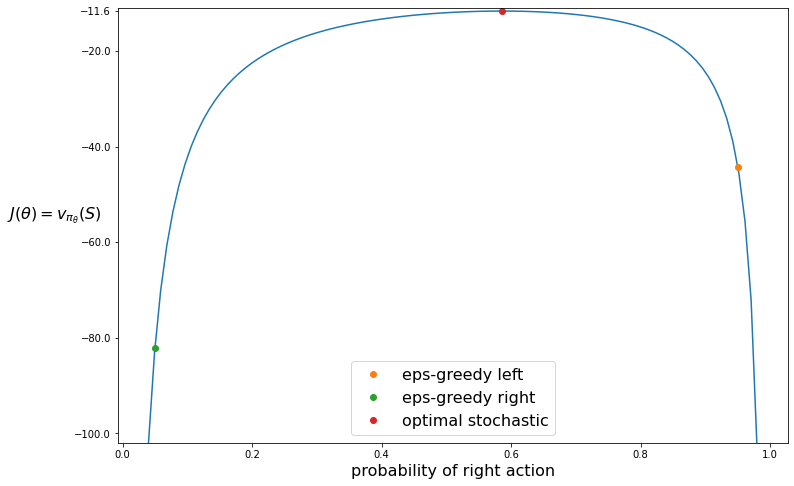### Gradient Monte Carlo for Value Function Approximation (RL S&B Example 9.1)

In this post we will implement Example 9.1 from Chapter 9 Reinforcement Learning (Sutton and Barto). This is an example of on-policy prediction with approximation which means that you try to approximate the value function for the policy you are following right now. We will be using Gradient Monte Carlo for approximating $v_\pi(s)$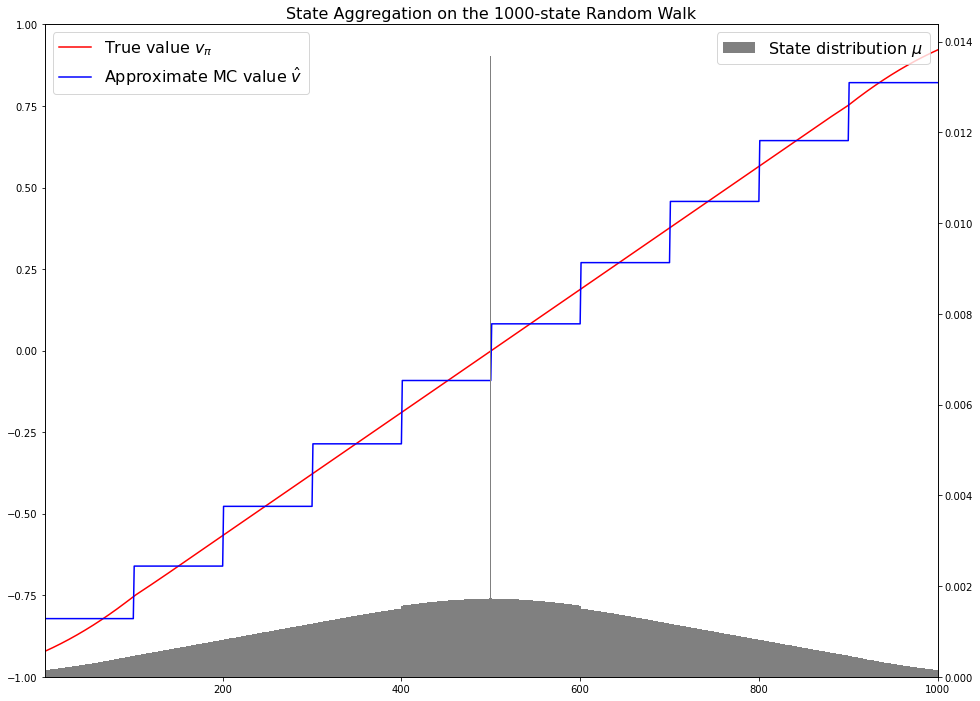### Vectorising the Bellman equations (RL S&B Examples 3.5, 3.8)

In this blog post we will reproduce Examples 3.5 and 3.8 in Reinforcement Learning (Sutton and Barto) involving the Bellman equation. This post presumes a basic understanding of reinforcement learning in particular policy and value and will just outline the theory that is needed in order to implement the examples.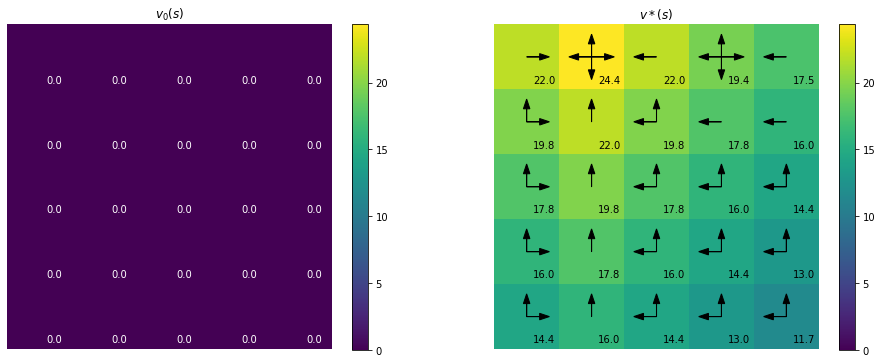## nlp

### How to generate subtitles with the Whisper API in Python

In this tutorial we will learn how to use the Whisper API to generate subtitles for a video. We will generate subtitles for the opening of A Star Is Born (1937), an early colour movie that has been frequently remade.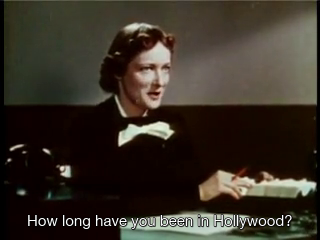### Transformer Part 2: Train a Translation Model

In Part 1 of the tutorial we learned how to how build a transformer model. Now it is time to put it into action. There are lots of things we can do with a transformer but we will start off with a machine translation task to translate from Spanish to English.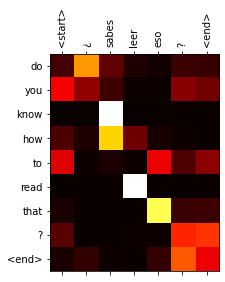### Transformer Part 1: Build

Since its introduction the Transformer architecture has become very influential and has had successes in many tasks not just in NLP but in other areas like vision. This tutorial is inspired by the approach in The Annotated Transformer and uses text directly quoted from the paper to explain the code.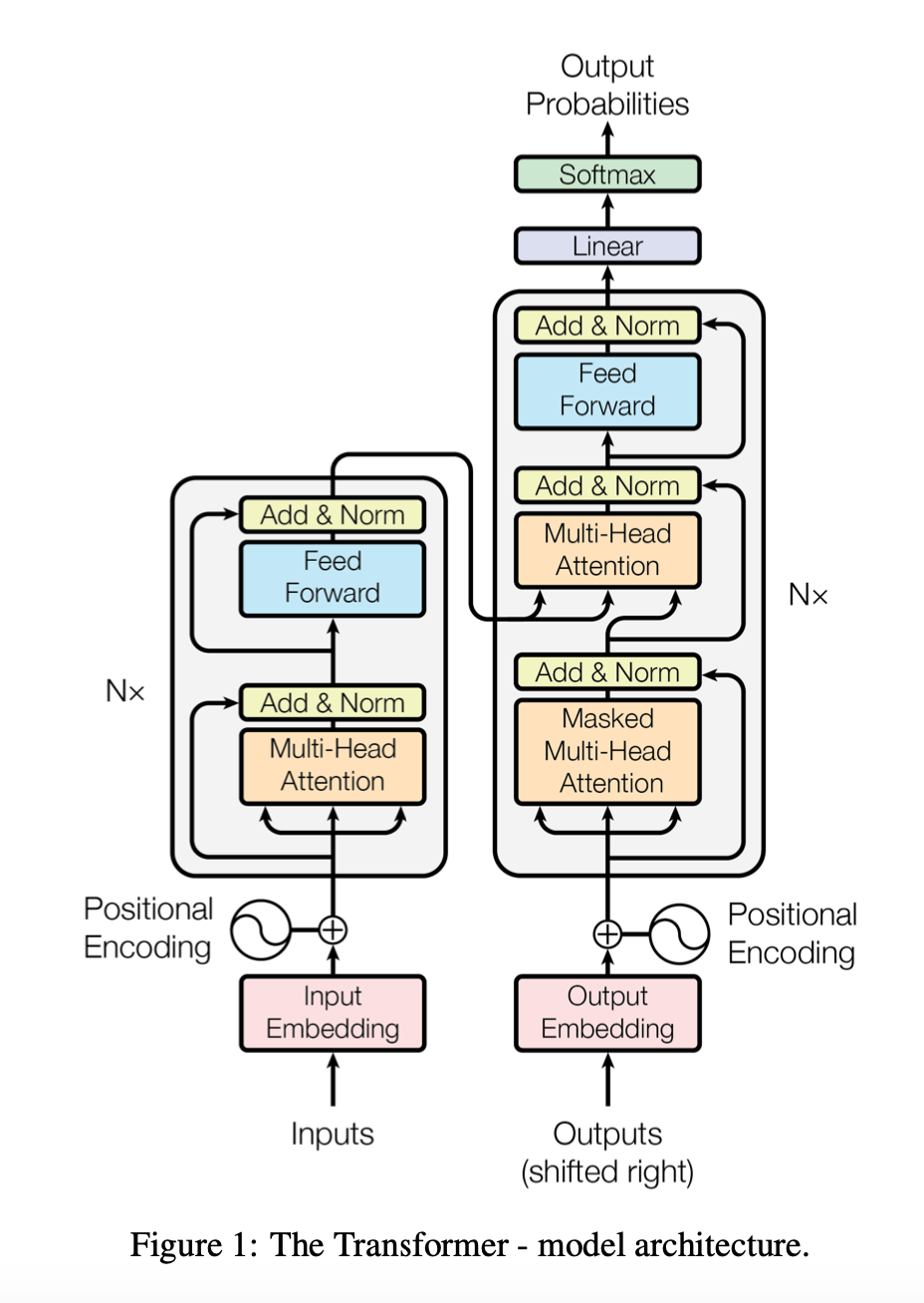### Veg2vec - word embeddings for plant-based foods with health benefits

One of the most exciting uses of machine learning is scientific discovery. Sometimes long before a discovery is officially made people have written about similar ideas and one could speculate what might have happened if some dots had been connected sooner. This is exactly what the mat2vec model (code, paper) seeks to do with the help of AI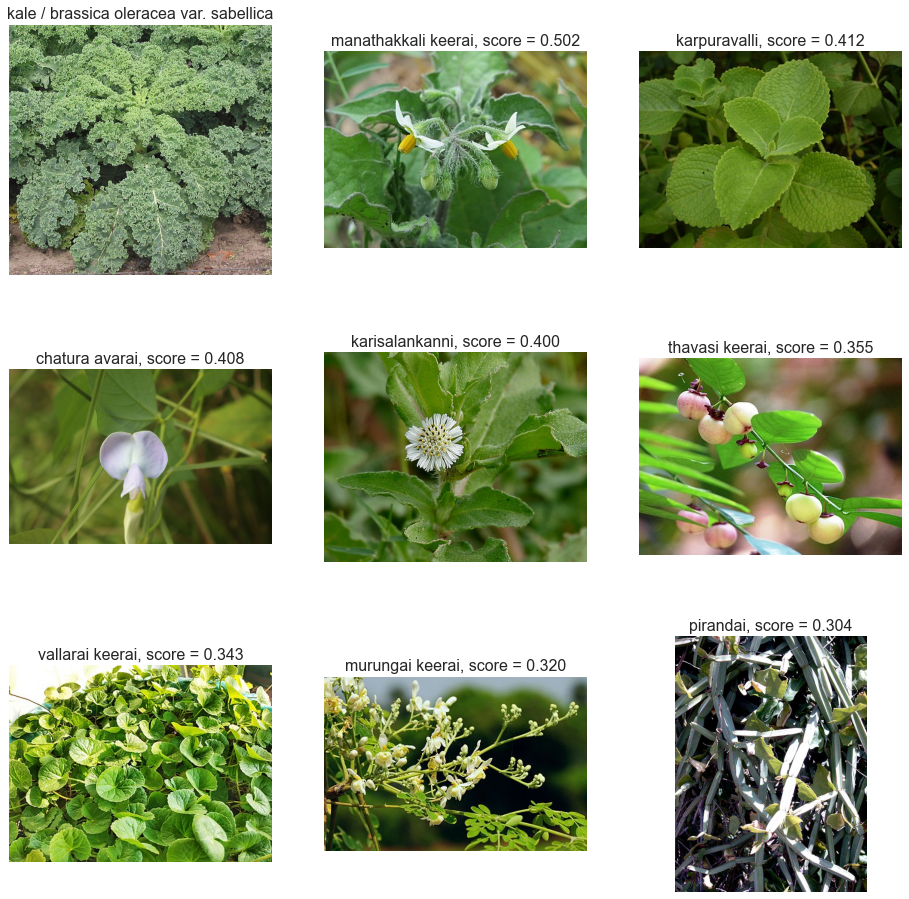### Padding in PyTorch and TensorFlow embedding layers

When batching inputs for sequence models you often have sequences of variable sizes and you need to pad some of the inputs so that you can input them as a single tensor. For example here is a pair of lines in a dialogue from Twelfth Night Act 2, Scene 4 which are of variable length as represented here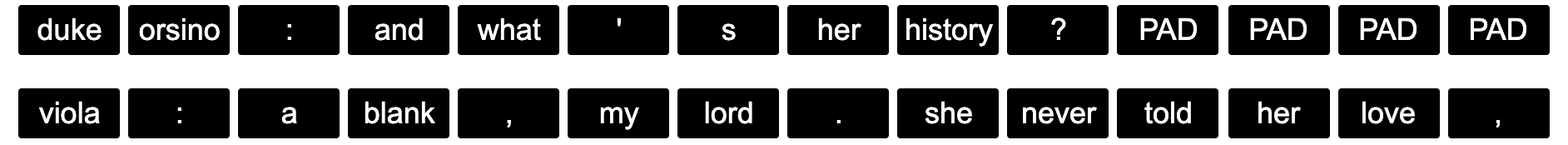However you don’t want the pad locations to influence the weight updates. In this post we will learn how PyTorch and TensorFlow approach this via their respective embedding layers.

## cv

### Generating crops from images

This post explains how to implement a function to generate a crop of a particular object from an image given the position of the centroid of the object. The images below show an example use case: cropping all the individual oranges from an image.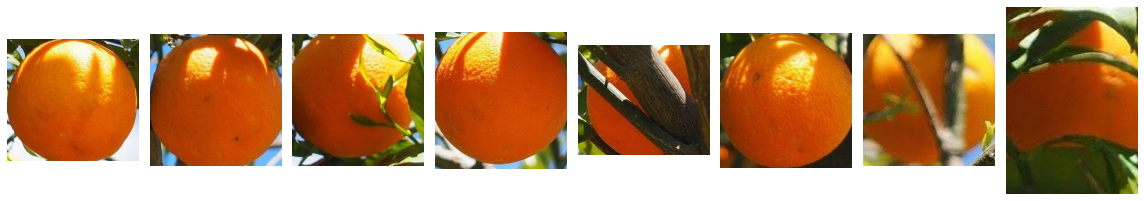### Non-maximum suppression (NMS)

This blogpost contains a quick practical introduction to a popular post-processing clustering algorithm used in object detection models. The code used to generate the figures and the demo can be found in this Colab notebook.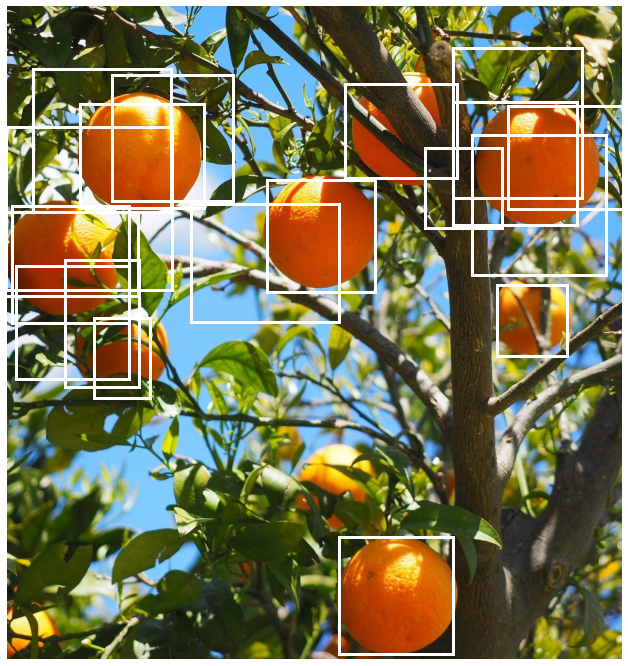### Vectorizing Intersection over Union (IoU)

Supporting batched data is an important requirement for a deep learning pipeline to enable time efficient model training. Often when writing a function, the easiest way to start is to first handle one element and then use a for loop over all the elements in the batch.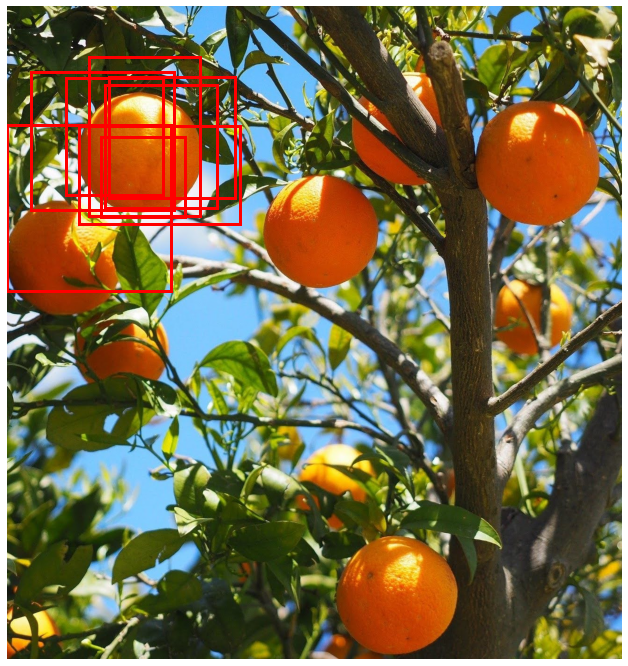### Converting Camera Conventions

When working with computer vision models we frequently need to know information about the camera that was used to generate the image. This includes information about the position of the camera in the world coordinate system as well as the intrinsic properties of the camera such as the focal length.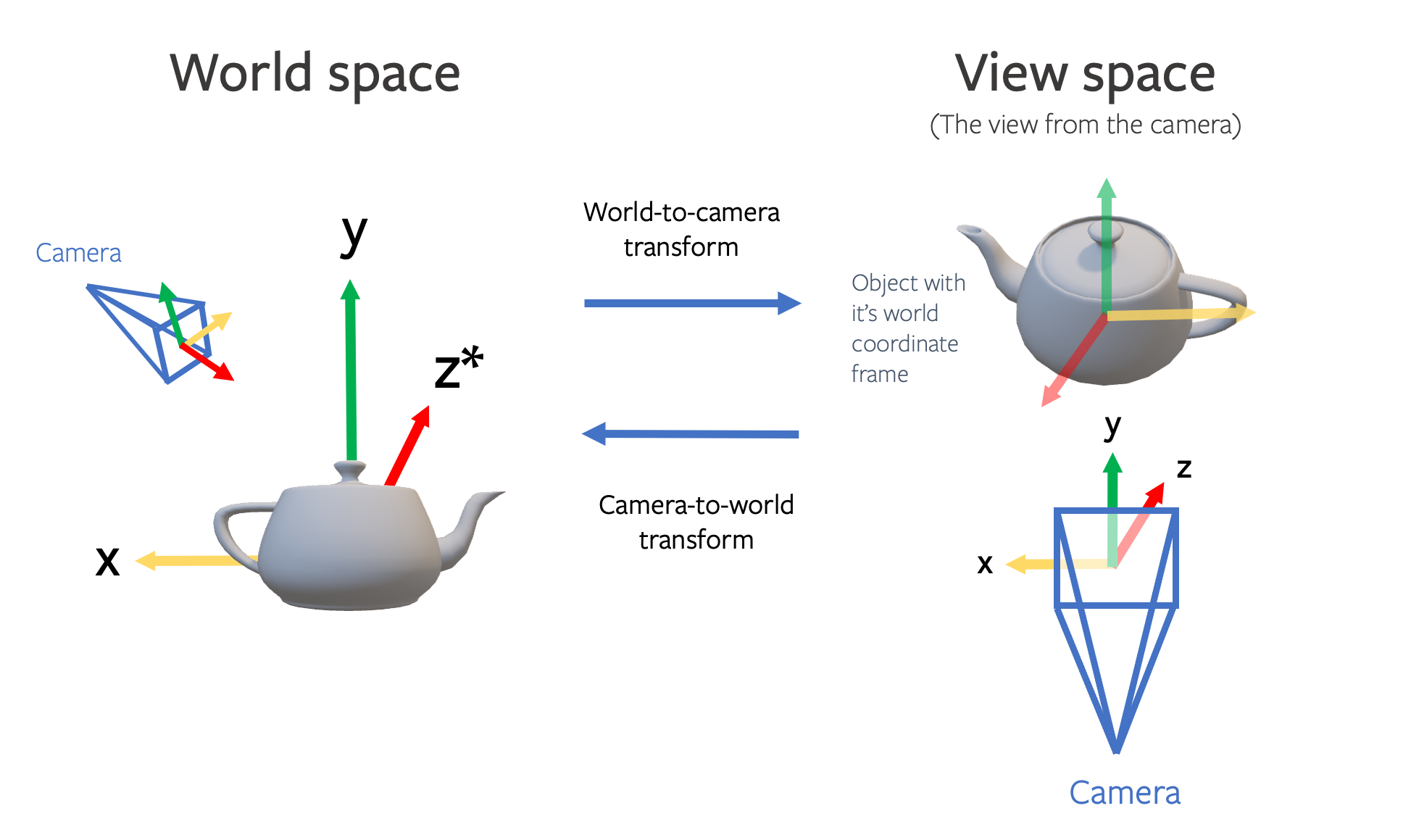### Plotting bounding boxes for object detection

When working with detection models in a pipeline you often need to visualize the bounding boxes to sanity check the predictions. Bounding boxes can be defined in different ways so this is also useful to confirm the convention being used.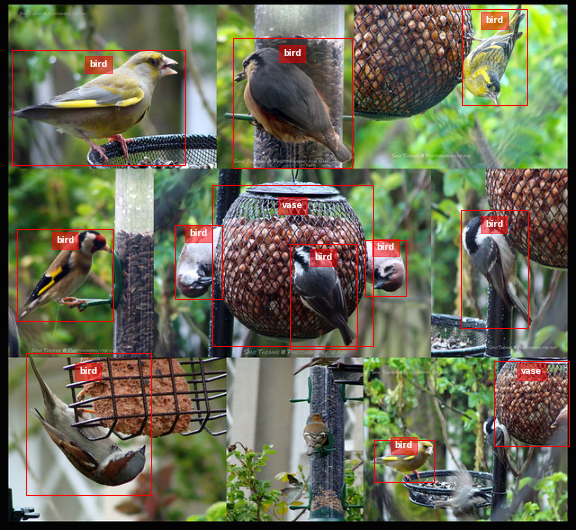## detection

### Generating crops from images

This post explains how to implement a function to generate a crop of a particular object from an image given the position of the centroid of the object. The images below show an example use case: cropping all the individual oranges from an image.### Non-maximum suppression (NMS)

This blogpost contains a quick practical introduction to a popular post-processing clustering algorithm used in object detection models. The code used to generate the figures and the demo can be found in this Colab notebook.### Vectorizing Intersection over Union (IoU)

Supporting batched data is an important requirement for a deep learning pipeline to enable time efficient model training. Often when writing a function, the easiest way to start is to first handle one element and then use a for loop over all the elements in the batch.### Plotting bounding boxes for object detection

When working with detection models in a pipeline you often need to visualize the bounding boxes to sanity check the predictions. Bounding boxes can be defined in different ways so this is also useful to confirm the convention being used.## sampling

### Speeding Up Diffusion Sampling

An important disadvantage with diffusion models is that a large number forward passes - typically 1000 or more - are needed to generate samples, making inference slow and expensive. In this post we will look at a couple of different methods that have been developed to speed up inference.### Spherical Interpolation

$\newcommand{\vv}{\mathbf{v}}$ In machine learning applications you sometimes want to interpolate vectors in a normalised latent space such as when interpolating between two images in a generative model. An appropriate method for doing this is spherical interpolation. In this post we will derive the formula for this method and show how it differs from linear interpolation.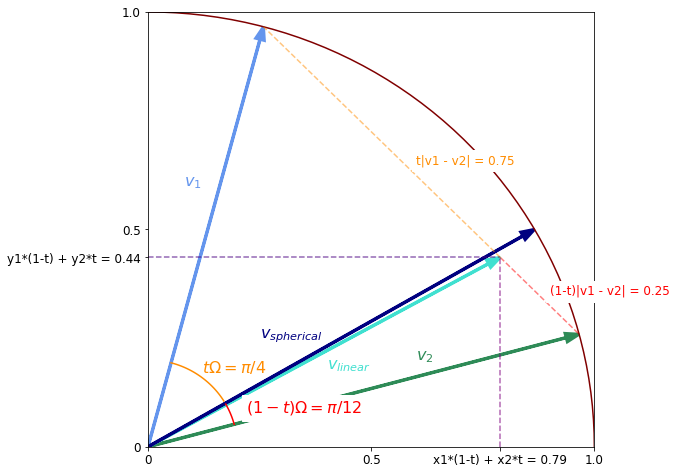### A Quick Introduction to Importance Sampling

You might have encountered the term importance sampling in a machine learning paper. This post provides a quick hands-on introduction to this topic. Hopefully after reading it you will have know understand how to use this technique in practice.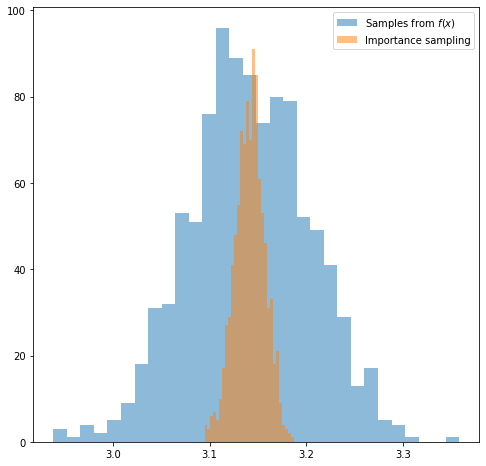### Furthest Point Sampling

I have come across an algorithm named furthest point sampling or sometimes farthest point sampling a few times in deep learning papers dealing with point cloud data. However there is a lack of resources online explaining what this algorithm actually does. Here I will try to explain it with reference to this CUDA implementation. Along the way we will also see how to implement it in an efficient parallelised way.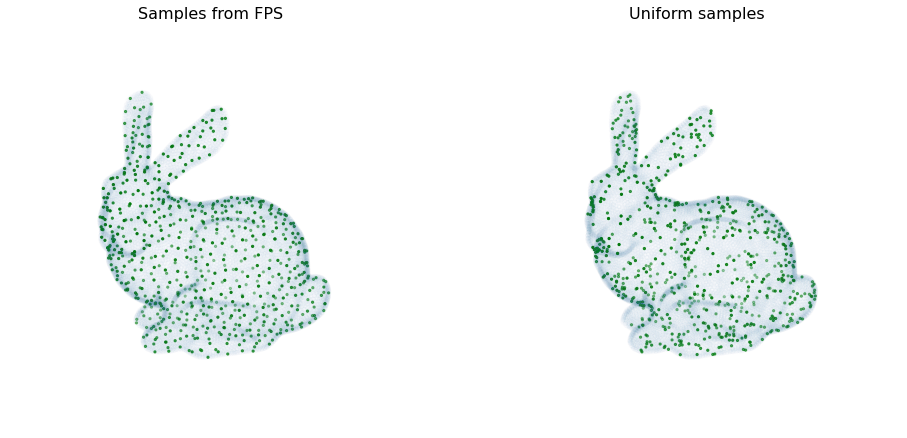## diffusion

### How to create non-square images with DALL-E 2

The DALL-E API from OpenAI is a very powerful image generation tool. However one limitation is that it only outputs square images. In this tutorial we will learn how to generate images of arbitrary dimension such as portraits and landscapes.### Speeding Up Diffusion Sampling

An important disadvantage with diffusion models is that a large number forward passes - typically 1000 or more - are needed to generate samples, making inference slow and expensive. In this post we will look at a couple of different methods that have been developed to speed up inference.### An Introduction to Diffusion

In this blog we will learn about the basic diffusion-based generative model introduced in Denoising Diffusion Probabilistic Models (DDPM). This post will cover the basics at a relatively high level but along the way there will be optional exercises that will help you to delve deeper into the mathematical details.### A quick overview of GLIDE

You have probably come across DALL-E 2 a large-scale image generation model from OpenAI. If not, you can read about it in their blog. DALL-E 2 uses a class of models known as diffusion models. The DALL-E 2 paper does not go into a lot of detail about the model architecture since it mostly extends an earlier architecture GLIDE. This article provides a brief introduction to the GLIDE architecture.## tensorflow

### Distributed BatchNorm in TensorFlow

In this post we will see an example of how BatchNorm works when running distributed training with TensorFlow using TPUs and tf.keras.layers. This post assumes you know BatchNormalisation works.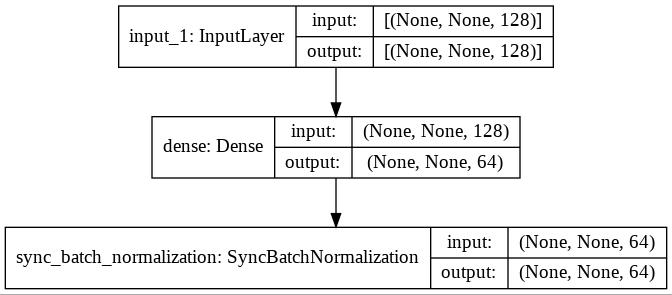### Cosine Learning Rate Decay

In this post we will introduce the key hyperparameters involved in cosine decay and take a look at how the decay part can be achieved in TensorFlow and PyTorch. In a subsequent blog we will look at how to add restarts.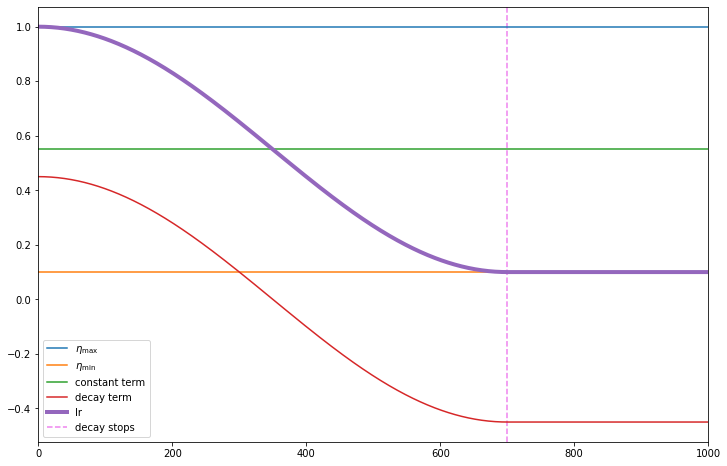### Padding in PyTorch and TensorFlow embedding layers

When batching inputs for sequence models you often have sequences of variable sizes and you need to pad some of the inputs so that you can input them as a single tensor. For example here is a pair of lines in a dialogue from Twelfth Night Act 2, Scene 4 which are of variable length as represented hereHowever you don’t want the pad locations to influence the weight updates. In this post we will learn how PyTorch and TensorFlow approach this via their respective embedding layers.

## text2image

### Speeding Up Diffusion Sampling

An important disadvantage with diffusion models is that a large number forward passes - typically 1000 or more - are needed to generate samples, making inference slow and expensive. In this post we will look at a couple of different methods that have been developed to speed up inference.### An Introduction to Diffusion

In this blog we will learn about the basic diffusion-based generative model introduced in Denoising Diffusion Probabilistic Models (DDPM). This post will cover the basics at a relatively high level but along the way there will be optional exercises that will help you to delve deeper into the mathematical details.### A quick overview of GLIDE

You have probably come across DALL-E 2 a large-scale image generation model from OpenAI. If not, you can read about it in their blog. DALL-E 2 uses a class of models known as diffusion models. The DALL-E 2 paper does not go into a lot of detail about the model architecture since it mostly extends an earlier architecture GLIDE. This article provides a brief introduction to the GLIDE architecture.## pytorch

### Cosine Learning Rate Decay

In this post we will introduce the key hyperparameters involved in cosine decay and take a look at how the decay part can be achieved in TensorFlow and PyTorch. In a subsequent blog we will look at how to add restarts.### Padding in PyTorch and TensorFlow embedding layers

When batching inputs for sequence models you often have sequences of variable sizes and you need to pad some of the inputs so that you can input them as a single tensor. For example here is a pair of lines in a dialogue from Twelfth Night Act 2, Scene 4 which are of variable length as represented hereHowever you don’t want the pad locations to influence the weight updates. In this post we will learn how PyTorch and TensorFlow approach this via their respective embedding layers.

## optimization

### Distributed BatchNorm in TensorFlow

In this post we will see an example of how BatchNorm works when running distributed training with TensorFlow using TPUs and tf.keras.layers. This post assumes you know BatchNormalisation works.### Cosine Learning Rate Decay

In this post we will introduce the key hyperparameters involved in cosine decay and take a look at how the decay part can be achieved in TensorFlow and PyTorch. In a subsequent blog we will look at how to add restarts.## metrics

### Implementing the Fréchet Inception distance

A popular metric for evaluating image generation models is the Fréchet Inception Distance (FID). Like the Inception score, it is computed on the embeddings from an Inception model. But unlike the Inception score, it makes use of the true images as well as the generated ones. In the post we will learn how to implement it in PyTorch.### Vectorizing Intersection over Union (IoU)

Supporting batched data is an important requirement for a deep learning pipeline to enable time efficient model training. Often when writing a function, the easiest way to start is to first handle one element and then use a for loop over all the elements in the batch.## transformer

### Transformer Part 2: Train a Translation Model

In Part 1 of the tutorial we learned how to how build a transformer model. Now it is time to put it into action. There are lots of things we can do with a transformer but we will start off with a machine translation task to translate from Spanish to English.### Transformer Part 1: Build

Since its introduction the Transformer architecture has become very influential and has had successes in many tasks not just in NLP but in other areas like vision. This tutorial is inspired by the approach in The Annotated Transformer and uses text directly quoted from the paper to explain the code.## attention

### Transformer Part 2: Train a Translation Model

In Part 1 of the tutorial we learned how to how build a transformer model. Now it is time to put it into action. There are lots of things we can do with a transformer but we will start off with a machine translation task to translate from Spanish to English.### Transformer Part 1: Build

Since its introduction the Transformer architecture has become very influential and has had successes in many tasks not just in NLP but in other areas like vision. This tutorial is inspired by the approach in The Annotated Transformer and uses text directly quoted from the paper to explain the code.## how-to

### How to create non-square images with DALL-E 2

The DALL-E API from OpenAI is a very powerful image generation tool. However one limitation is that it only outputs square images. In this tutorial we will learn how to generate images of arbitrary dimension such as portraits and landscapes.### How to generate subtitles with the Whisper API in Python

In this tutorial we will learn how to use the Whisper API to generate subtitles for a video. We will generate subtitles for the opening of A Star Is Born (1937), an early colour movie that has been frequently remade.## augmentation

### Generating crops from images

This post explains how to implement a function to generate a crop of a particular object from an image given the position of the centroid of the object. The images below show an example use case: cropping all the individual oranges from an image.## vae

### Variational Auto-Encoder - Part 1 / Introduction

Consider this painting by Vincent Van Gogh known as “Wheatfield with Crows”. Suppose you want to learn a probability distribution over Van Gogh’s paintings. What the computer or a deep learning algorithm sees when it looks at this painting is a grid of RGB pixel intensities. So one approach might be to model the data as a sequence of individual pixels. Yet that is manifestly not how the artist has constructed the painting.## normalization

### Distributed BatchNorm in TensorFlow

In this post we will see an example of how BatchNorm works when running distributed training with TensorFlow using TPUs and tf.keras.layers. This post assumes you know BatchNormalisation works.## algorithms

### Strassen’s algorithm for matrix multiplication

This is the first of a planned series of blogs covering background topics for DeepMind’s AlphaTensor paper. In this post we will cover Strassen’s algorithm for matrix multiplication.## auto-regressive

### PixelCNN

The PixelRNN family of auto-regressive architectures consists of models that learn to generate images a pixel at a time. In this post we will focus on the PixelCNN model which is based on the same principles but uses convolutional layers instead of recurrent layers. It is simpler to implement and once we have grasped the core concepts, it is straightforward to understand the recurrent versions.## llm

### How to generate subtitles with the Whisper API in Python

In this tutorial we will learn how to use the Whisper API to generate subtitles for a video. We will generate subtitles for the opening of A Star Is Born (1937), an early colour movie that has been frequently remade.